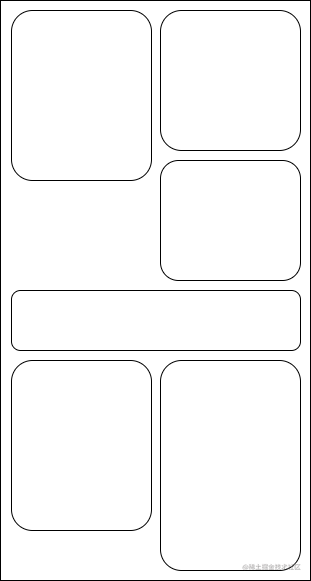## 微信小程序实现可以截断的瀑布流组件``````<view class="container" style="height:{{height}}px;">
<view wx:for="{{list}}" wx:key="index" style="{{item.style}}" class="wrapper">
<abstract item="{{item}}"/>
</view>
</view>
<view wx:if="{{tmp}}" class="computed-zone">
<view class="wrapper">
<abstract item="{{tmp}}"/>
</view>
</view>

`wrapper` 容器是一个绝对定位的包裹元素，`wrapper` 容器里面需要放置需要实际渲染的组件，为了灵活性更高一点，我把这个渲染组件设置成了虚拟节点，在使用组件的时候可以指定实际渲染的自定义组件。

1. 如果元素的渲染宽度跟容器一样，那么就可以判断这个元素沾满一整行，需要将包裹容器 `wrapper` 设置为一整行的宽度；
2. 如果不满足1条件，那么就需要基于左右元素的总高度，将 `wrapper` 放在左侧或者右侧。

``````{
// 将 setData Promise 化，方便使用
\$setData(data) {
return new Promise(resolve => {
this.setData(data, () => {
resolve();
});
});
},
// 获取元素的渲染尺寸
getRect(item) {
return this.\$setData({
tmp: item,
}).then(() => {
return new Promise((resolve, reject) => {
const query = this.createSelectorQuery(); // 注意要使用 this，不要再使用 wx 前缀了
query.select('.computed-zone .wrapper').boundingClientRect();
query.exec(ret => {
if (ret) {
resolve(ret);
} else {
}
});
});
});
},
// 添加元素，内部使用
let tick = this.tick;
return this.getRect(item).then(rect => {
if (tick !== this.tick) {
return Promise.reject('tick');
}
const { margin } = this.data;
let { height, width } = rect;
const windowWidth = this.sysInfo.windowWidth;
let [ leftTotal, rightTotal ] = this.height; // leftTotal 左侧栏高度，rightTotal 右侧栏高度，
let marginPx = this.sysInfo.getPx(margin);
let style = '';

if (Math.abs(width - windowWidth) < 3) {
// 占满屏幕宽度
style = `left:0;top:\${ Math.max(leftTotal, rightTotal) }px;width:100%;`;
leftTotal = rightTotal = Math.max(leftTotal + height, rightTotal + height);
} else if (rightTotal < leftTotal) {
// 放入右边
style = `right:\${ marginPx }px;top:\${ rightTotal }px;`;
rightTotal += height;
} else {
// 放入左边
style = `left:\${ marginPx }px;top:\${ leftTotal }px;`;
leftTotal += height;
}

const { list = [] } = this.data;
const targetKey = `list[\${list.length}]`; // 利用直接操作数组下标的方式来触发数组修改，性能有很大提升
this.height = [leftTotal, rightTotal]; // 记录最新的左右侧高度
return this.\$setData({
[targetKey]: {
data: item,
style,
},
height: Math.max(leftTotal, rightTotal),
});
});
},
// 实际添加元素使用，自建Promise队列，保证顺序一致
let pending = this.pending || Promise.resolve();
return this.pending = pending.then(() => {
}).catch(err => {
console.error(err);
this.pending = null;
throw err;
});
},
clear() {
this.tick = tick++;
this.height = [0, 0];
this.pending = null;
this.setData({
list: [],
height: 0,
});
},
}